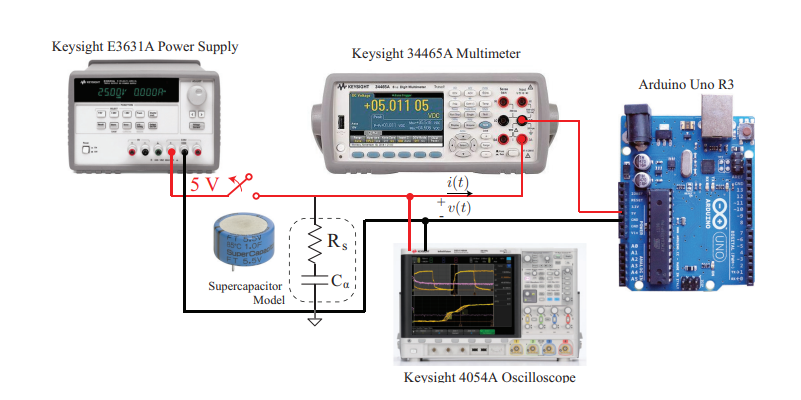Conference Paper

# Supercapacitor Fractional-Order Model Discharging from Polynomial Time-Varying Currents

By
Freeborn T.J.
Elwakil A.S.
Allagui A.

Fractional-order models of supercapacitors are advantageous in that they have fewer terms, offering simpler expressions to accurately describe the transient characteristics of these devices than integer-order models. When evaluating the discharge characteristics of supercapacitors, a constant current is often considered which does necessarily represent real-world applications. In this work, the voltage discharging expressions of a fractional-order model of a supercapacitor to time-varying polynomial discharging currents are presented using simulations to highlight the different cases. In addition, the parameters of a commercial supercapacitor are determined from experimental data when constant, linear, and quadratic discharging currents are assumed to highlight the implications that the current discharge waveform has on the estimated device parameters. © 2018 IEEE.